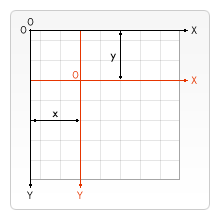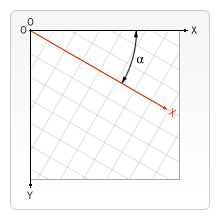# 变形 Transformations

## 状态的保存和恢复

save()

restore()

save 和 restore 方法是用来保存和恢复 canvas 状态的，都没有参数。Canvas 的状态就是当前画面应用的所有样式和变形的一个快照。

Canvas 状态存储在栈中，每当save()方法被调用后，当前的状态就被推送到栈中保存。一个绘画状态包括：

### save 和 restore 的应用例子我们尝试用这个连续矩形的例子来描述 canvas 的状态栈是如何工作的。

function draw() {
var ctx = document.getElementById('canvas').getContext('2d');

ctx.fillRect(0,0,150,150);   // 使用默认设置绘制一个矩形
ctx.save();                  // 保存默认状态

ctx.fillStyle = '#09F'       // 在原有配置基础上对颜色做改变
ctx.fillRect(15,15,120,120); // 使用新的设置绘制一个矩形

ctx.save();                  // 保存当前状态
ctx.fillStyle = '#FFF'       // 再次改变颜色配置
ctx.globalAlpha = 0.5;
ctx.fillRect(30,30,90,90);   // 使用新的配置绘制一个矩形

ctx.restore();               // 重新加载之前的颜色状态
ctx.fillRect(45,45,60,60);   // 使用上一次的配置绘制一个矩形

ctx.restore();               // 加载默认颜色配置
ctx.fillRect(60,60,30,30);   // 使用加载的配置绘制一个矩形
}


## 移动 Translatingtranslate(x, y)

translate方法接受两个参数。*x *是左右偏移量，y 是上下偏移量，如右图所示。

### translate 的例子

function draw() {
var ctx = document.getElementById('canvas').getContext('2d');
for (var i = 0; i < 3; i++) {
for (var j = 0; j < 3; j++) {
ctx.save();
ctx.fillStyle = 'rgb(' + (51 * i) + ', ' + (255 - 51 * i) + ', 255)';
ctx.translate(10 + j * 50, 10 + i * 50);
ctx.fillRect(0, 0, 25, 25);
ctx.restore();
}
}
}


## 旋转 Rotatingrotate(angle)

### rotate 的例子

function draw() {
const ctx = document.getElementById("canvas").getContext("2d");

// left rectangles, rotate from canvas origin
ctx.save();
// blue rect
ctx.fillStyle = "#0095DD";
ctx.fillRect(30, 30, 100, 100);
ctx.rotate((Math.PI / 180) * 25);
// grey rect
ctx.fillStyle = "#4D4E53";
ctx.fillRect(30, 30, 100, 100);
ctx.restore();

// right rectangles, rotate from rectangle center
// draw blue rect
ctx.fillStyle = "#0095DD";
ctx.fillRect(150, 30, 100, 100);

ctx.translate(200, 80); // translate to rectangle center
// x = x + 0.5 * width
// y = y + 0.5 * height
ctx.rotate((Math.PI / 180) * 25); // rotate
ctx.translate(-200, -80); // translate back

// draw grey rect
ctx.fillStyle = "#4D4E53";
ctx.fillRect(150, 30, 100, 100);
}


## 缩放 Scaling

scale(x, y)

scale方法可以缩放画布的水平和垂直的单位。两个参数都是实数，可以为负数，x 为水平缩放因子，y 为垂直缩放因子，如果比 1 小，会缩小图形，如果比 1 大会放大图形。默认值为 1，为实际大小。

### scale 的例子

function draw() {
var ctx = document.getElementById('canvas').getContext('2d');

// draw a simple rectangle, but scale it.
ctx.save();
ctx.scale(10, 3);
ctx.fillRect(1, 10, 10, 10);
ctx.restore();

// mirror horizontally
ctx.scale(-1, 1);
ctx.font = '48px serif';
ctx.fillText('MDN', -135, 120);
}


## 变形 Transforms

transform(a, b, c, d, e, f)

$\left\left[ \begin\left\{array\right\}\left\{ccc\right\} a & c & e \ b & d & f \ 0 & 0 & 1 \end\left\{array\right\} \right\right]$

a (m11)

b(m12)

c(m21)

d(m22)

e(dx)

f(dy)

setTransform(a, b, c, d, e, f)

resetTransform()

### transform/setTransform 的例子

function draw() {
var ctx = document.getElementById('canvas').getContext('2d');

var sin = Math.sin(Math.PI/6);
var cos = Math.cos(Math.PI/6);
ctx.translate(100, 100);
var c = 0;
for (var i=0; i <= 12; i++) {
c = Math.floor(255 / 12 * i);
ctx.fillStyle = "rgb(" + c + "," + c + "," + c + ")";
ctx.fillRect(0, 0, 100, 10);
ctx.transform(cos, sin, -sin, cos, 0, 0);
}

ctx.setTransform(-1, 0, 0, 1, 100, 100);
ctx.fillStyle = "rgba(255, 128, 255, 0.5)";
ctx.fillRect(0, 50, 100, 100);
}